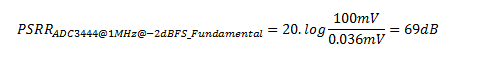# Measuring PSR in an ADC

Other Parts Discussed in Post: ADC3444

In order to avoid power-supply noise compromising signal integrity in a signal-chain analog-to-digital converter (ADC), it is important to measure the power-supply rejection (PSR) of the ADC.  This blog post will focus on explaining the techniques needed for this measurement, and describe how to derive the PSR of the ADC.

ADCs require one or more power supplies whose sensitivity may affect the data acquisition of the ADC if precautions are not taken. Power-supply sensitivity is independent from clocking jitter issues, which are fairly well understood at this time.  We will only focus on deriving power-supply sensitivity in this post, using the high-speed TI ADC3444 ADC as an example.  The ADC3444 is a quad-channel 14bit 125Mbps ADC designed for high-performance multichannel applications.

Looking at the data sheet the ADC3444 has two distinct power supplies: a 1.8V analog supply and a 1.8V digital supply.  The data sheet provides the following information in the electrical characteristics (Section 7.7) section and in the application section (Section 11).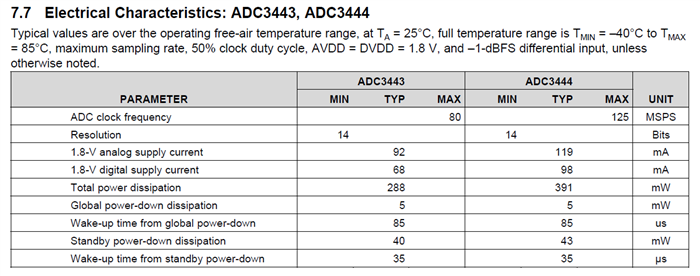When evaluating the performance of an ADC, the ADC has the advantage of being its own digitizer. We are interested in what will happen to the fast Fourier transform (FFT) while digitizing a single-tone signal and adding a noise tone to the ADC power-supply pins. In order to simplify, we will not worry about the supply noise, but will send a sine wave superimposed on the DC power-supply voltage. We can achieve this superimposition using a power amplifier, as shown in Figure 1.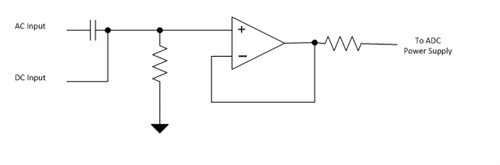Figure 1: Power amplifier simplified schematic

Note that the DC gain is set to 1V/V. The small isolation resistor is used at the output to help prevent instability in the amplifier caused by capacitive loading.

At this point, we will only be looking at measuring the power-supply characteristic of the ADC at a single frequency, leaving a full PSRR-over-frequency plot for later. Since the ADC has very high analog bandwidth, we expect the PSRR of the analog supply to be flat to high frequency – or at least beyond the maximum frequency of interest when designing a power supply. In the case of the ADC3444, the analog input bandwidth is 540MHz. Note that adding any bypass capacitance on the power-supply pin will improve the measured PSR at high frequency, as any high frequency will be bypassed by the capacitor.

The test configuration, shown in figure 2, does not make any distinction between the analog supply pin (AVDD) and the digital supply pin (DVDD).  To isolate possible interactions between the AVDD and DVDD supplies, the measurement procedure introduces a noise tone on a single supply at a time.  The decoupling on each supply follows the ADC3444 datasheet recommendation. The thirteen AVDD pins will use each a 0.1µF, X7R capacitor for a total of 1.3µF.  Similarly, the four DVDD supply pins will each have a 0.22µF, for a total of 0.88µF on the DVDD supply.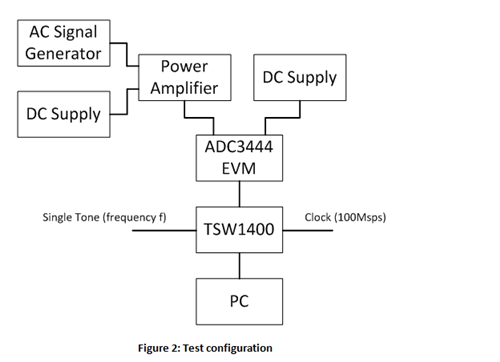Figure 2: Test configuration

Figure 3 shows the results for ADC3444 power supplies.  Each chart below is the Fast Fourier Transform (FFT) of the analog signal.  The FFT is the decomposition of the signal as a sum of sinewave.  Simply put, the charts show the frequency content of the signal.  The x-axis is thus the frequency, with the y-axis representing the magnitude of each sinewave.  In the example below, we use a 100MHz clock, resulting in the acquisition bandwidth of 50MHz.

The signal fed to the ADC is a 19.8MHz with a magnitude of -2dBFS (dB Below Full Scale).  This is shown in figure 3, which is the reference signal with no noise tone.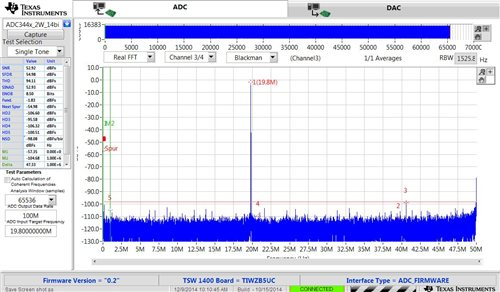Figure 3: ADC3444 AVDD reference FFT

To evaluate the PSR of the ADC, the following procedure is devised for each supply:

1-      Connect the amplifier to the power supply being evaluated [Here this will be either the AVDD or the DVDD]

2-      Connect the DC-power supply to the other power supplies.  [This DC power supply is a clean power supply and has been selected for its low noise characteristics]

3-      Turn on the noise tone and capture the FFT.  [The noise tone has been selected to maximize the disturbance on the supply pins under test, while still respecting the supply tolerance.]

Figure 4 shows the same signal tone into the ADC, but this time adding a noise tone in the AVDD power supply pin.  Since all other conditions are equal, any degradation on the FTT can be correlated to the addition of the noise tone on the AVDD supply.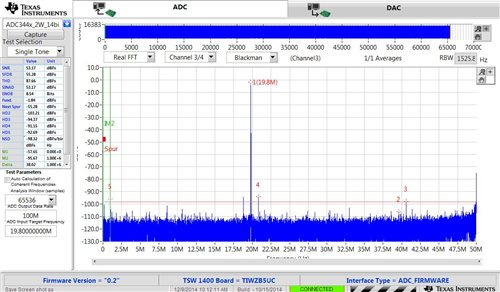Figure 4: ADC3444 AVDD response to 100mVpp 1MHz sinewave superimposed to the 1.8V

We then repeat the same experiment on the DVDD supply pin and obtain the chart shown in figure 5.  This time the noise tone is only present on the DVDD supply.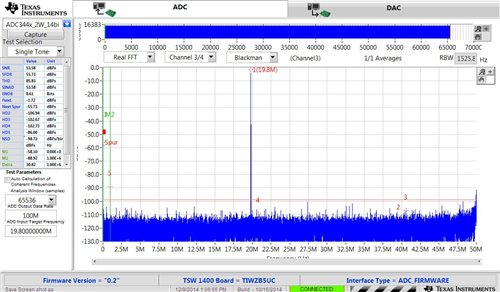Figure 5: ADC3444 DVDD response to 100mVpp 1MHz sinewave superimposed to the 1.8V

Notice that for the AVDD supply (Figure 4), three additional spurs have appeared: at 1MHz, 18.8MHz and 20.8MHz. The first tone is to be expected, as the noise tone gets added to the acquired spectrum. The two other undesired tones are exactly 1MHz to the right and to the left, symmetrical to the center frequency.

For the DVDD supply, the only difference between Figure 3 and Figure 5 is a new spur at 1MHz.

Figure 6 summarizes the location of the spurs. Note that the spurs’ amplitudes are intended to be purely descriptive and not quantitative.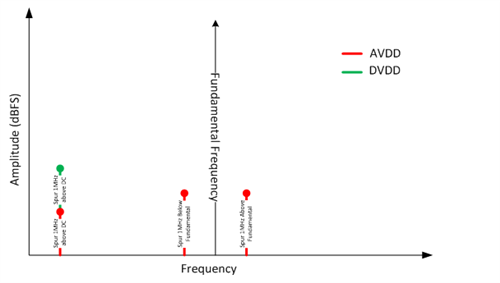Figure 6: Spurs location for AVDD and DVDD supplies

Now that we have an initial measurement, we still need to interpret it to be able to extract the desired PSRR spec. The 100mV AC signal, called a disturbance signal, ensures that it will be sufficiently large enough to stick out of the ADC noise floor, while not so large that it will violate the operating voltage range on both AVDD and DVDD.

Let’s use Figures 6 and 7 to help us interpret the result and translate the dB below full scale (dBFS) to a PSRR specification. Say that the fundamental amplitude is -2dBFS. Since the ADC3444 data sheet, in figure 7, tells us that 0dBFS (or full scale) is 2Vpp, we can convert the dBFS into Vpp. This is possible with Equation 1 below: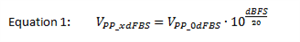Applying this to the -2dBFS fundamental, the differential voltage swing at the ADC’s input is: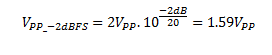We can now convert the dBFS measurement from the ADC FFT result into a signal that we can compare to the AC input signal, and thus calculate how much rejection the ADC power supply has. The 100mV signal on the AVDD supply has an impact of -95dBFS for a -2dBFS signal. The data sheet tells us that 0dBFS is 2Vpp (see Figure 5), so the -95dBFS can be calculated by: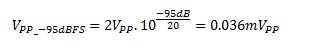The PSRR can then be calculated with Equation 2:

Plugging the numbers into Equation 2 gives us the PSRR (Power Supply Rejection Ratio) for a -2dBFS fundamental amplitude and a 1MHz disturber signal on the power supply.